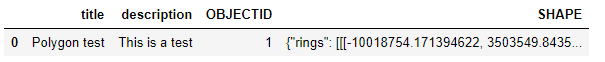# Add a polygon to a Spatially Enabled Dataframe

1325
1
10-26-2020 11:14 AMNew Contributor II

Hi,

Sorry if this is a basic question but I can't seem to crack it. I am creating a Polygon feature from a list of xy values and want to add it to a Spatially Enabled Dataframe. How could I make a SEDF that holds the following polygon?

``````import pandas as pd
from arcgis.geometry import Polygon
from arcgis.features import SpatialDataFrame

shape = {
"rings": [[[-90.0, 30.0], [-91.0, 30.0], [-90.0, 31.0]]],
"spatialreference" : {"wkid" : 4326}}

polygonFeature = Polygon(shape)

print(polygonFeature.is_valid)

df = pd.DataFrame()‍‍‍‍‍‍‍‍‍‍‍‍‍``````

The polygon created from the values is valid, I'm just not sure how I could add it to an SEDF and then append additional polygons.

Thanks for any help!

1 Solution

Accepted SolutionsbyEsri Contributor

Hi Matthew Thompson‌,

You can use the following code to create a spatial dataframe from the shape:

``````from arcgis import GIS
from arcgis.features import SpatialDataFrame, Feature, FeatureSet
import pandas as pd
from arcgis import geometry

shape = {
"rings": [[[-90.0, 30.0], [-91.0, 30.0], [-90.0, 31.0]]],
"spatialreference" : {"wkid" : 4326}}

polygonFeature = Polygon(shape)

polygon_new = Feature(geometry=polygonFeature, attributes={"title": "Polygon test",
"description": "This is a test",})
#Create a featureset to access spatial dataframe
polygon_fset = FeatureSet(features = [polygon_new],
geometry_type="Polygon",
spatial_reference={'latestWkid': 4326, 'wkid': 102100})
polygon_fset.sdf‍‍‍‍‍‍‍‍‍‍‍‍‍‍‍‍‍‍``````-----------------------------------------------------------------------------------------------------------------------------------------------byEsri Contributor

Hi Matthew Thompson‌,

You can use the following code to create a spatial dataframe from the shape:

``````from arcgis import GIS
from arcgis.features import SpatialDataFrame, Feature, FeatureSet
import pandas as pd
from arcgis import geometry

shape = {
"rings": [[[-90.0, 30.0], [-91.0, 30.0], [-90.0, 31.0]]],
"spatialreference" : {"wkid" : 4326}}

polygonFeature = Polygon(shape)

polygon_new = Feature(geometry=polygonFeature, attributes={"title": "Polygon test",
"description": "This is a test",})
#Create a featureset to access spatial dataframe
polygon_fset = FeatureSet(features = [polygon_new],
geometry_type="Polygon",
spatial_reference={'latestWkid': 4326, 'wkid': 102100})
polygon_fset.sdf‍‍‍‍‍‍‍‍‍‍‍‍‍‍‍‍‍‍``````-----------------------------------------------------------------------------------------------------------------------------------------------